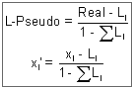# Component Scaling in Mixture Designs

Three scales are used to transform the component levels to a convenient scale for analysis. The original scale used to input the component levels is the “Actual”. These are converted to the “Real” scale, with each component re-scaled so that the total is 1.0. In the real scale, the components are then fractions of each component in the mixture. If the total of the components is set to 80% then the “Real” values are the actual values divided by 0.80.

For analysis, the “Pseudo” scale is preferred. In the pseudo scale each component is rescaled to be a fraction of the “active” part of the mixture. L-Pseudo is the default scale, but U-Pseudo will be offered if collinearity is lower in this scale. (See mathematical description below.)

To illustrate, suppose that a three-component nut mixture will always contain at least 50% peanuts, at least 15% pecans and at least 5% cashews. Therefore, 70% of the nut mix is predetermined. We are going to do an experiment to determine the remaining 30%. In pseudo terms,

Peanut pseudo component = (% peanut – 50%) / 30%

Pecan pseudo component = (% pecan – 15%) / 30%

Cashew pseudo component = (% cashew – 5%) / 30%

If there are no lower limit constraints, the real and pseudo scales are identical.

After building the design you may change the scale via the Display Options menu.

## L-Pseudo vs U-Pseudo

Lower bounded pseudo values: the minimum value of each component becomes zero; the maximum value approaches one. How close it comes to one depends on the constraints. For a simplex the component minimums are zero and the maximums are one.Upper bounded pseudo values: the maximum value of each component becomes zero; the minimum value approaches one. How close it comes to one depends on the constraints. For an inverted simplex the component maximums are zero and the minimums are one.